# Chapter 9 Interfaces and Polymorphism Chapter 9 Interfaces

• Slides: 80Chapter 9 Interfaces and Polymorphism Chapter 9 Interfaces and Polymorphism 1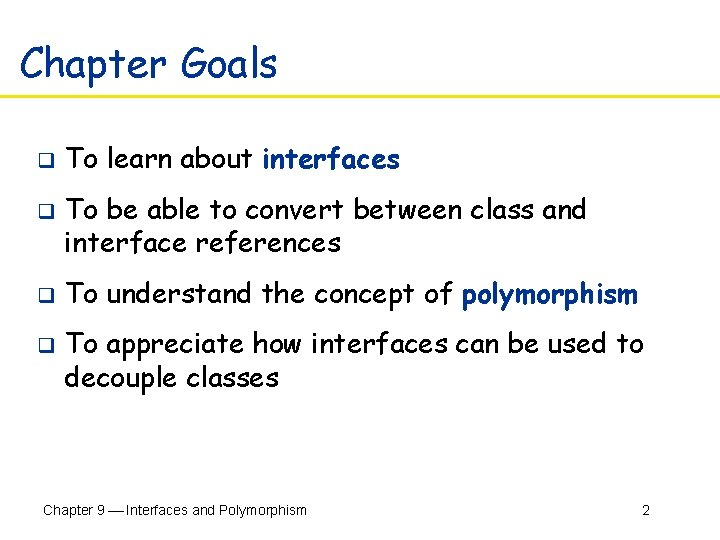Chapter Goals q q To learn about interfaces To be able to convert between class and interface references To understand the concept of polymorphism To appreciate how interfaces can be used to decouple classes Chapter 9 Interfaces and Polymorphism 2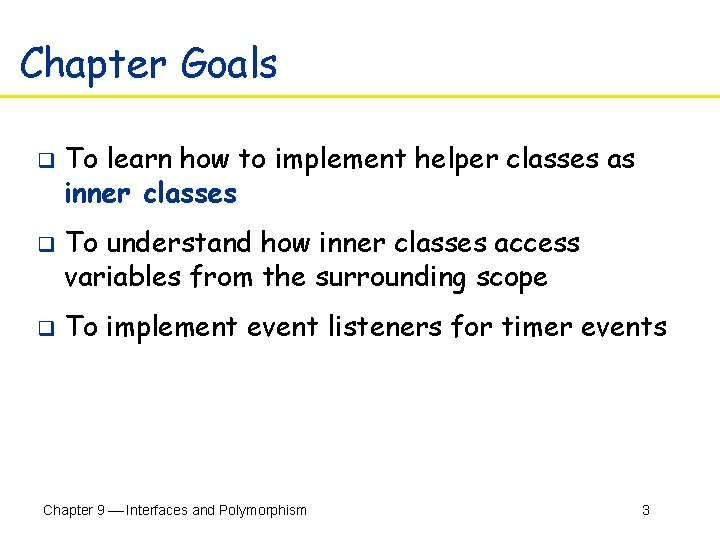Chapter Goals q q q To learn how to implement helper classes as inner classes To understand how inner classes access variables from the surrounding scope To implement event listeners for timer events Chapter 9 Interfaces and Polymorphism 3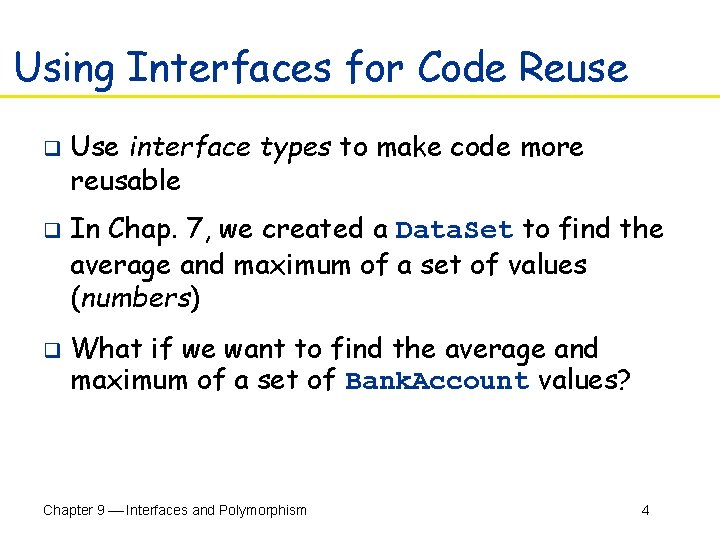Using Interfaces for Code Reuse q q q Use interface types to make code more reusable In Chap. 7, we created a Data. Set to find the average and maximum of a set of values (numbers) What if we want to find the average and maximum of a set of Bank. Account values? Chapter 9 Interfaces and Polymorphism 4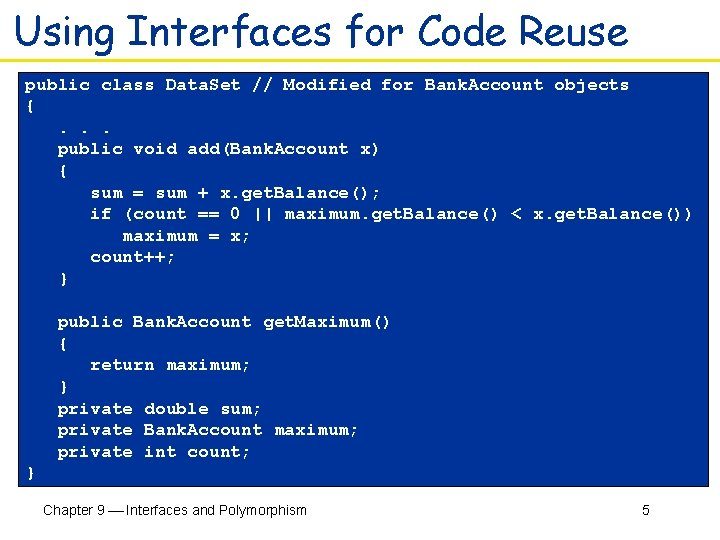Using Interfaces for Code Reuse public class Data. Set // Modified for Bank. Account objects { . . . public void add(Bank. Account x) { sum = sum + x. get. Balance(); if (count == 0 || maximum. get. Balance() < x. get. Balance()) maximum = x; count++; } public Bank. Account get. Maximum() { return maximum; } private double sum; private Bank. Account maximum; private int count; } Chapter 9 Interfaces and Polymorphism 5Using Interfaces for Code Reuse q Or suppose we wanted to find the Coin with the highest value among a set of coins. We would need to modify the Data. Set class again Chapter 9 Interfaces and Polymorphism 6Using Interfaces for Code Reuse public class Data. Set // Modified for Coin objects { . . . public void add(Coin x) { sum = sum + x. get. Value(); if (count == 0 || maximum. get. Value() < x. get. Value()) maximum = x; count++; } public Coin get. Maximum() { return maximum; } private double sum; private Coin maximum; private int count; } Chapter 9 Interfaces and Polymorphism 7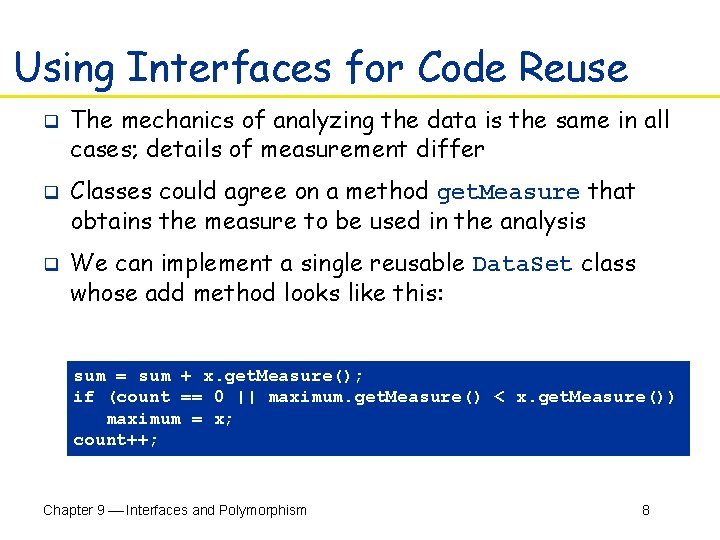Using Interfaces for Code Reuse q q q The mechanics of analyzing the data is the same in all cases; details of measurement differ Classes could agree on a method get. Measure that obtains the measure to be used in the analysis We can implement a single reusable Data. Set class whose add method looks like this: sum = sum + x. get. Measure(); if (count == 0 || maximum. get. Measure() < x. get. Measure()) maximum = x; count++; Chapter 9 Interfaces and Polymorphism 8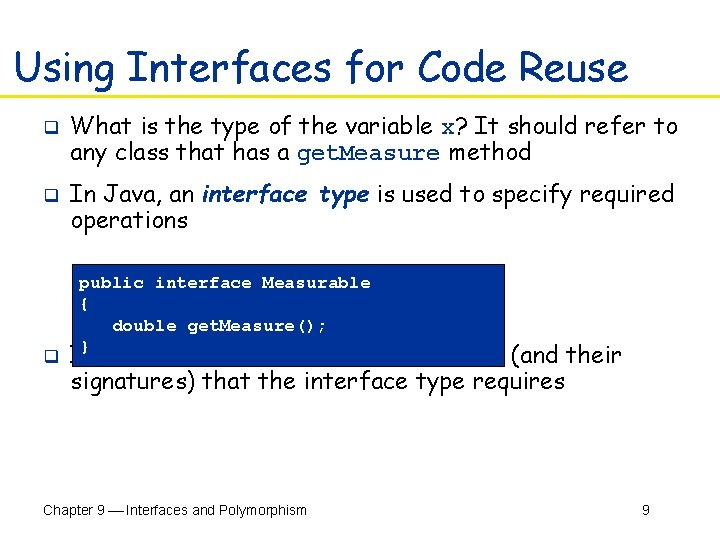Using Interfaces for Code Reuse q q q What is the type of the variable x? It should refer to any class that has a get. Measure method In Java, an interface type is used to specify required operations public interface Measurable { double get. Measure(); } Interface declaration lists all methods (and their signatures) that the interface type requires Chapter 9 Interfaces and Polymorphism 9Interfaces vs Classes q An interface type is similar to a class, but there are several important differences § All methods in an interface type are abstract (they do not have an implementation) § All methods in an interface type are automatically public § An interface type does not have instance fields Chapter 9 Interfaces and Polymorphism 10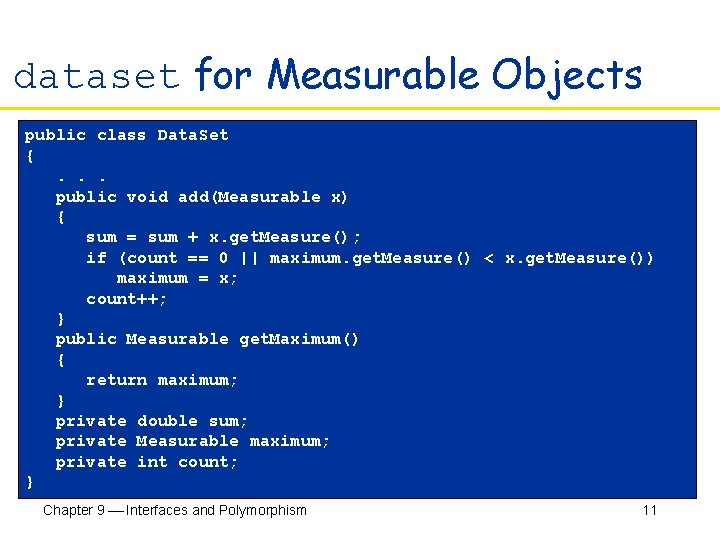dataset for Measurable Objects public class Data. Set { . . . public void add(Measurable x) { sum = sum + x. get. Measure(); if (count == 0 || maximum. get. Measure() < x. get. Measure()) maximum = x; count++; } public Measurable get. Maximum() { return maximum; } private double sum; private Measurable maximum; private int count; } Chapter 9 Interfaces and Polymorphism 11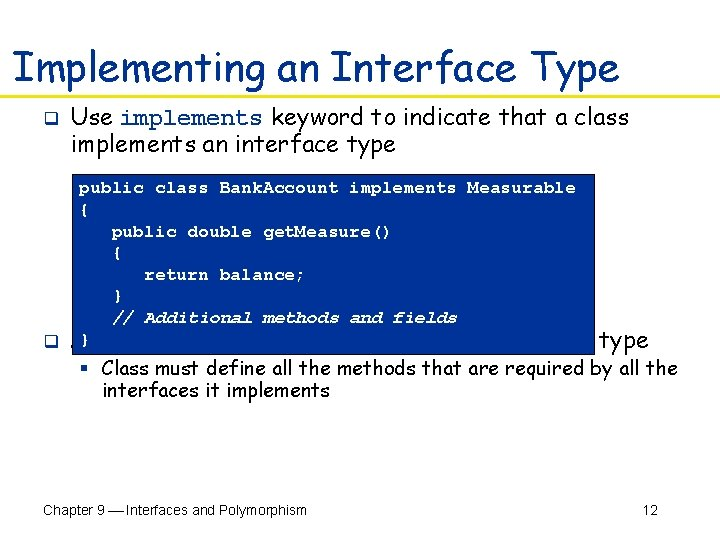Implementing an Interface Type q Use implements keyword to indicate that a class implements an interface type public class Bank. Account implements Measurable { public double get. Measure() { return balance; } // Additional methods and fields q A} class can implement more than one interface type § Class must define all the methods that are required by all the interfaces it implements Chapter 9 Interfaces and Polymorphism 12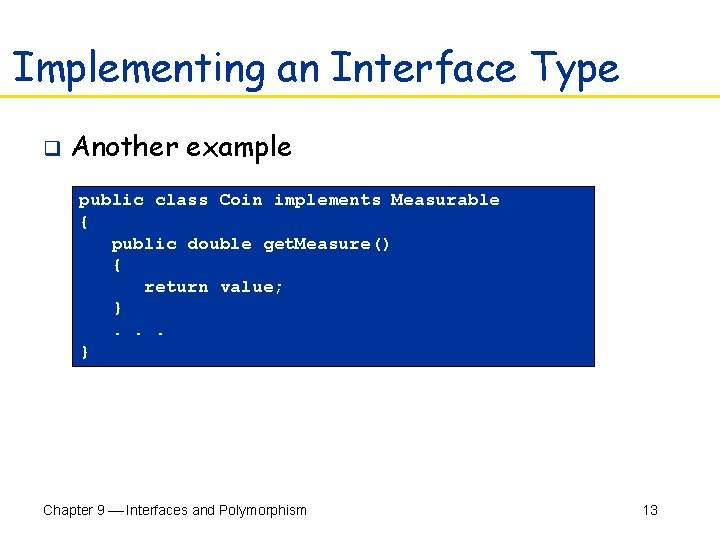Implementing an Interface Type q Another example public class Coin implements Measurable { public double get. Measure() { return value; } . . . } Chapter 9 Interfaces and Polymorphism 13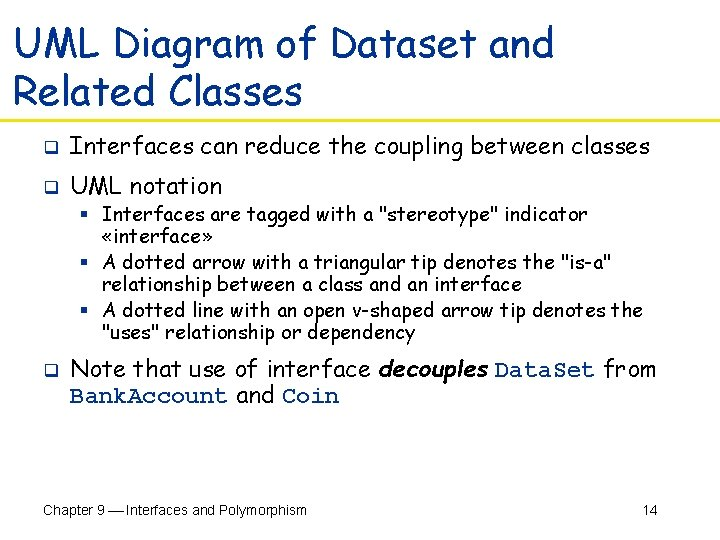UML Diagram of Dataset and Related Classes q Interfaces can reduce the coupling between classes q UML notation § Interfaces are tagged with a "stereotype" indicator «interface» § A dotted arrow with a triangular tip denotes the "is-a" relationship between a class and an interface § A dotted line with an open v-shaped arrow tip denotes the "uses" relationship or dependency q Note that use of interface decouples Data. Set from Bank. Account and Coin Chapter 9 Interfaces and Polymorphism 14UML Diagram of Dataset and Related Classes Figure 2: UML Diagram of Dataset Class and the Classes that Implement the Measurable Interface Chapter 9 Interfaces and Polymorphism 15Syntax 11. 1: Defining an Interface public interface Interface. Name { // method signatures } Example: public interface Measurable { double get. Measure(); } Purpose: To define an interface and its method signatures. The methods are automatically public. Chapter 9 Interfaces and Polymorphism 16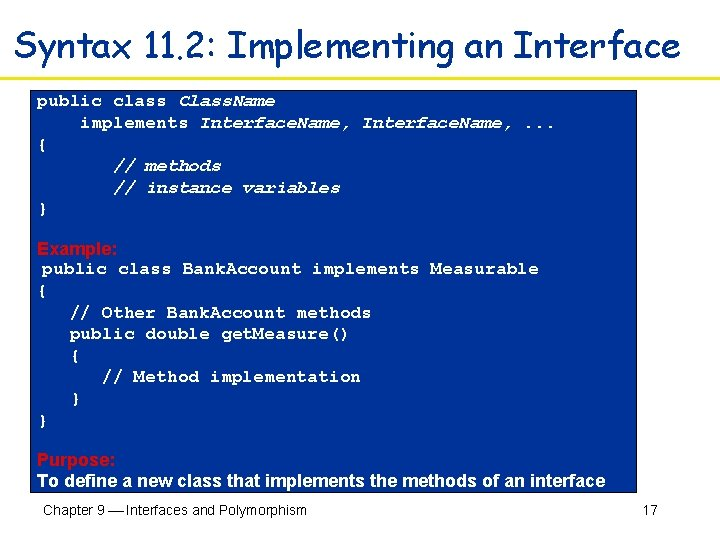Syntax 11. 2: Implementing an Interface public class Class. Name implements Interface. Name, . . . { // methods // instance variables } Example: public class Bank. Account implements Measurable { // Other Bank. Account methods public double get. Measure() { // Method implementation } } Purpose: To define a new class that implements the methods of an interface Chapter 9 Interfaces and Polymorphism 17File Data. Set. Tester. java 01: /** 02: This program tests the Data. Set class. 03: */ 04: public class Data. Set. Tester 05: { 06: public static void main(String[] args) 07: { 08: Data. Set bank. Data = new Data. Set(); 09: 10: bank. Data. add(new Bank. Account(0)); 11: bank. Data. add(new Bank. Account(10000)); 12: bank. Data. add(new Bank. Account(2000)); 13: 14: System. out. println("Average balance = " 15: + bank. Data. get. Average()); 16: Measurable max = bank. Data. get. Maximum(); 17: System. out. println("Highest balance = " 18: + max. get. Measure()); Continued… Chapter 9 Interfaces and Polymorphism 18File Data. Set. Tester. java 19: 20: Data. Set coin. Data = new Data. Set(); 21: 22: coin. Data. add(new Coin(0. 25, "quarter")); 23: coin. Data. add(new Coin(0. 1, "dime")); 24: coin. Data. add(new Coin(0. 05, "nickel")); 25: 26: System. out. println("Average coin value = " 27: + coin. Data. get. Average()); 28: max = coin. Data. get. Maximum(); 29: System. out. println("Highest coin value = " 30: + max. get. Measure()); 31: } 32: } Continued… Chapter 9 Interfaces and Polymorphism 19File Data. Set. Tester. java Output: Average balance = 4000. 0 Highest balance = 10000. 0 Average coin value = 0. 133333333 Highest coin value = 0. 25 Chapter 9 Interfaces and Polymorphism 20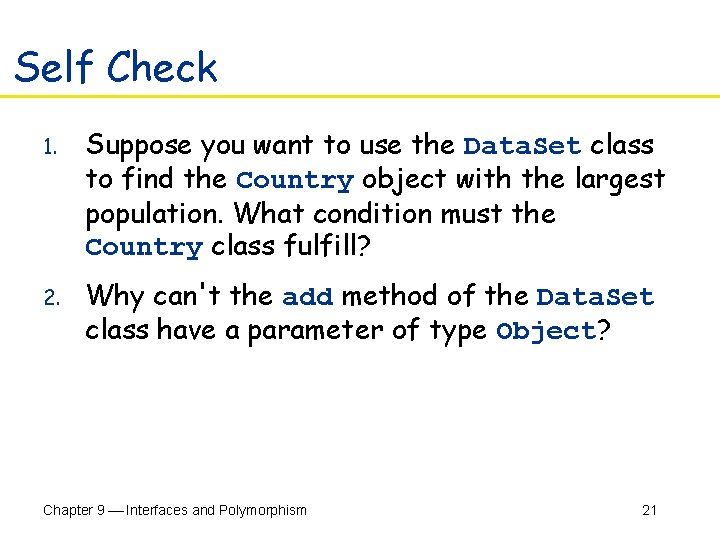Self Check 1. Suppose you want to use the Data. Set class to find the Country object with the largest population. What condition must the Country class fulfill? 2. Why can't the add method of the Data. Set class have a parameter of type Object? Chapter 9 Interfaces and Polymorphism 21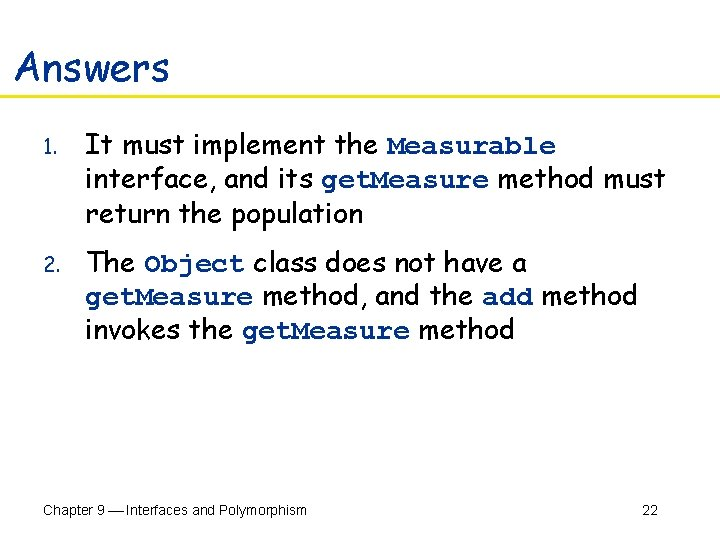Answers 1. It must implement the Measurable interface, and its get. Measure method must return the population 2. The Object class does not have a get. Measure method, and the add method invokes the get. Measure method Chapter 9 Interfaces and Polymorphism 22Converting Between Class and Interface Types q You can convert from a class type to an interface type, provided the class implements the interface q Bank. Account account = new Bank. Account(10000); Measurable x = account; // OK Coin dime = new Coin(0. 1, "dime"); Measurable x = dime; // Also OK Chapter 9 Interfaces and Polymorphism 23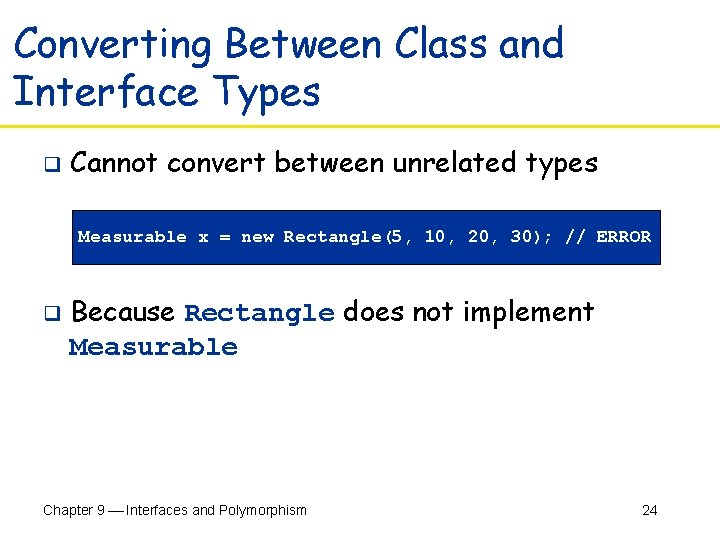Converting Between Class and Interface Types q Cannot convert between unrelated types Measurable x = new Rectangle(5, 10, 20, 30); // ERROR q Because Rectangle does not implement Measurable Chapter 9 Interfaces and Polymorphism 24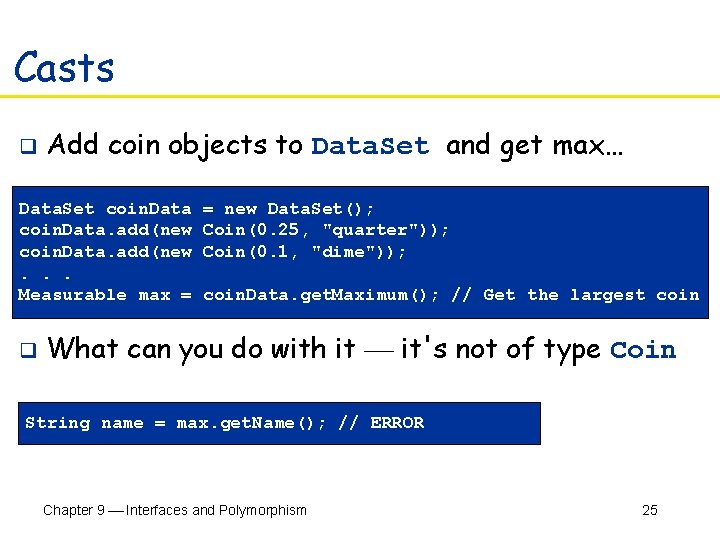Casts q Add coin objects to Data. Set and get max… Data. Set coin. Data = new Data. Set(); coin. Data. add(new Coin(0. 25, "quarter")); coin. Data. add(new Coin(0. 1, "dime")); . . . Measurable max = coin. Data. get. Maximum(); // Get the largest coin q What can you do with it it's not of type Coin String name = max. get. Name(); // ERROR Chapter 9 Interfaces and Polymorphism 25Casts q q q You need a cast to convert from an interface type to a class type You know it's a Coin, but the compiler does not, so apply a cast Coin max. Coin = (Coin) max; String name = max. Coin. get. Name(); If you are wrong and max is not a Coin, the compiler throws an exception (error) Chapter 9 Interfaces and Polymorphism 26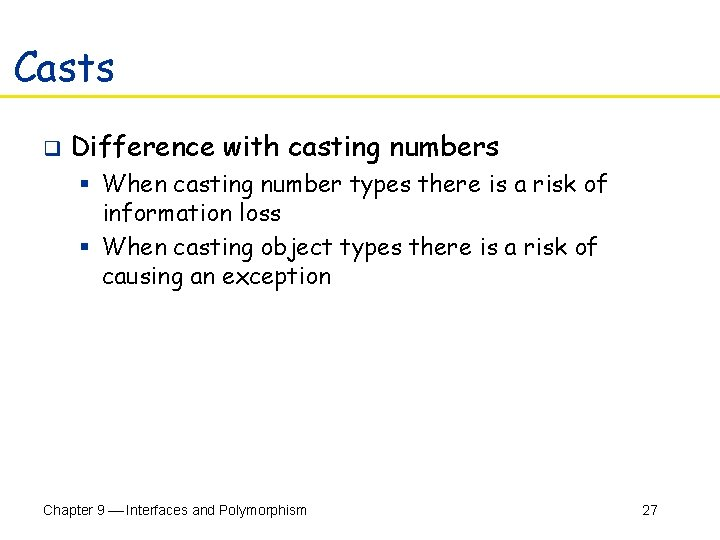Casts q Difference with casting numbers § When casting number types there is a risk of information loss § When casting object types there is a risk of causing an exception Chapter 9 Interfaces and Polymorphism 27Self Check 3. Can you use a cast (Bank. Account) x to convert a Measurable variable x to a Bank. Account reference? 4. If both Bank. Account and Coin implement the Measurable interface, can a Coin reference be converted to a Bank. Account reference? Chapter 9 Interfaces and Polymorphism 28Answers 3. Only if x actually refers to a Bank. Account object 4. No. A Coin reference can be converted to a Measurable reference, but if you attempt to cast that reference to a Bank. Account, an exception occurs. Chapter 9 Interfaces and Polymorphism 29Polymorphism q Interface variable holds reference to object of a class that implements the interface: Measurable x; x = new Bank. Account(10000); x = new Coin(0. 1, "dime"); Note that the object to which x refers doesn't have type Measurable the type of the object is some class that implements the Measurable interface Chapter 9 Interfaces and Polymorphism 30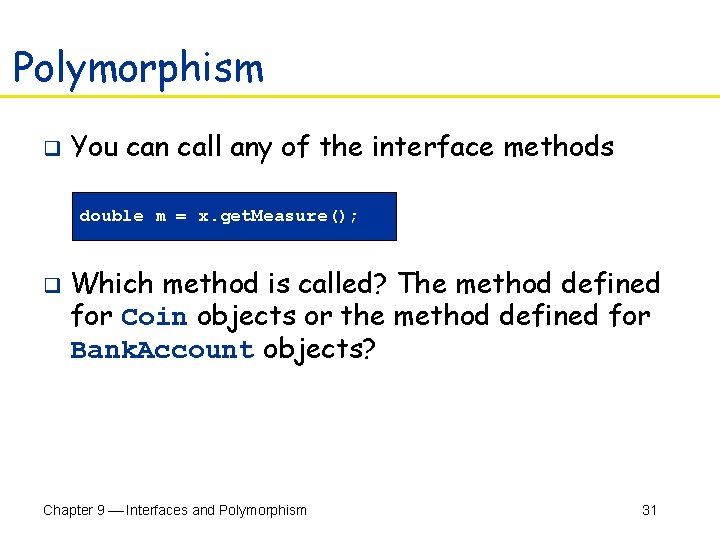Polymorphism q You can call any of the interface methods double m = x. get. Measure(); q Which method is called? The method defined for Coin objects or the method defined for Bank. Account objects? Chapter 9 Interfaces and Polymorphism 31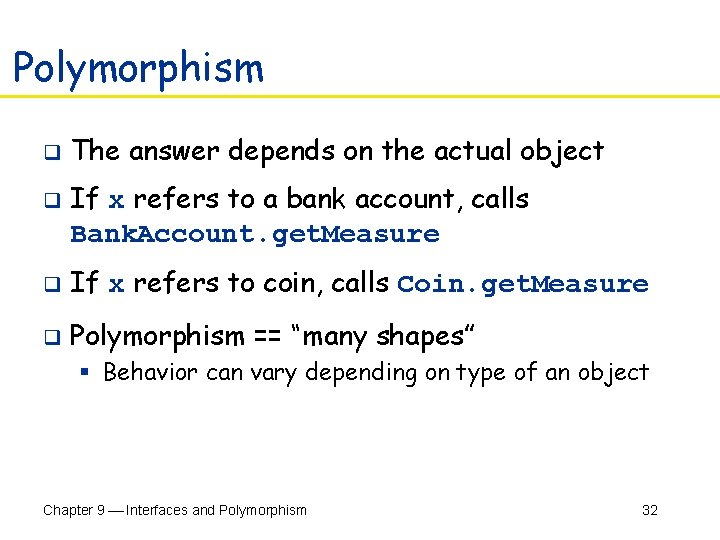Polymorphism q q The answer depends on the actual object If x refers to a bank account, calls Bank. Account. get. Measure q If x refers to coin, calls Coin. get. Measure q Polymorphism == “many shapes” § Behavior can vary depending on type of an object Chapter 9 Interfaces and Polymorphism 32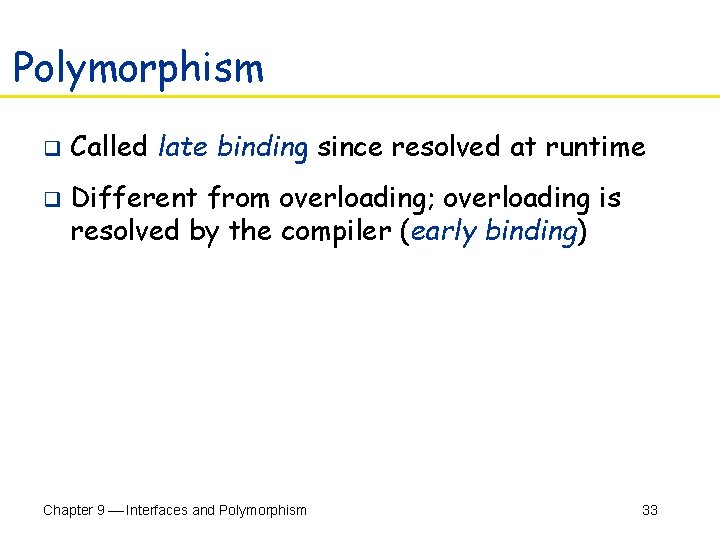Polymorphism q q Called late binding since resolved at runtime Different from overloading; overloading is resolved by the compiler (early binding) Chapter 9 Interfaces and Polymorphism 33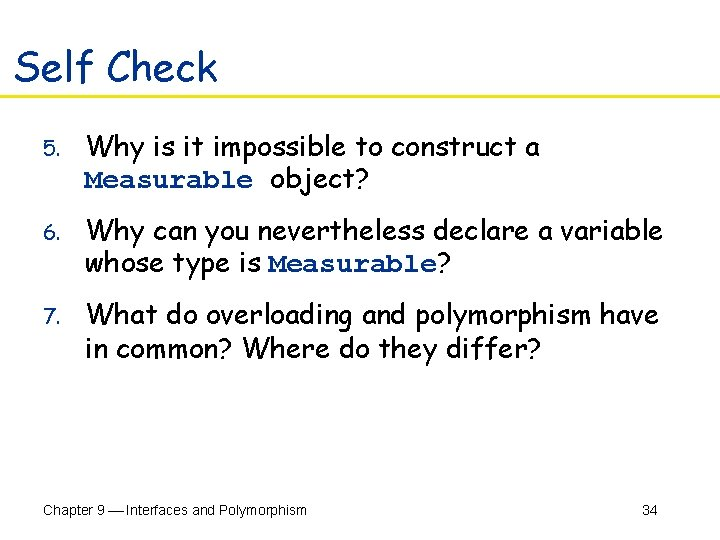Self Check 5. Why is it impossible to construct a Measurable object? 6. Why can you nevertheless declare a variable whose type is Measurable? 7. What do overloading and polymorphism have in common? Where do they differ? Chapter 9 Interfaces and Polymorphism 34Answers 5. Measurable is an interface and interfaces have no fields and no method implementations 6. That variable never refers to a Measurable “object” it refers to an object of some class that implements the Measurable interface Chapter 9 Interfaces and Polymorphism 35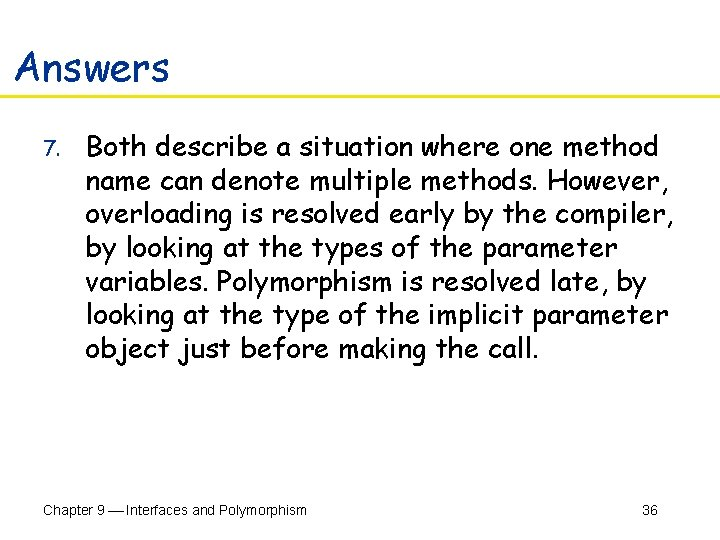Answers 7. Both describe a situation where one method name can denote multiple methods. However, overloading is resolved early by the compiler, by looking at the types of the parameter variables. Polymorphism is resolved late, by looking at the type of the implicit parameter object just before making the call. Chapter 9 Interfaces and Polymorphism 36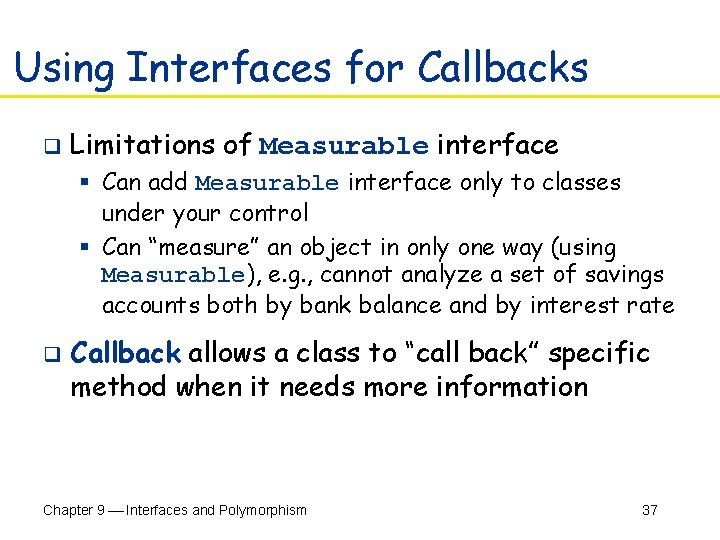Using Interfaces for Callbacks q Limitations of Measurable interface § Can add Measurable interface only to classes under your control § Can “measure” an object in only one way (using Measurable), e. g. , cannot analyze a set of savings accounts both by bank balance and by interest rate q Callback allows a class to “call back” specific method when it needs more information Chapter 9 Interfaces and Polymorphism 37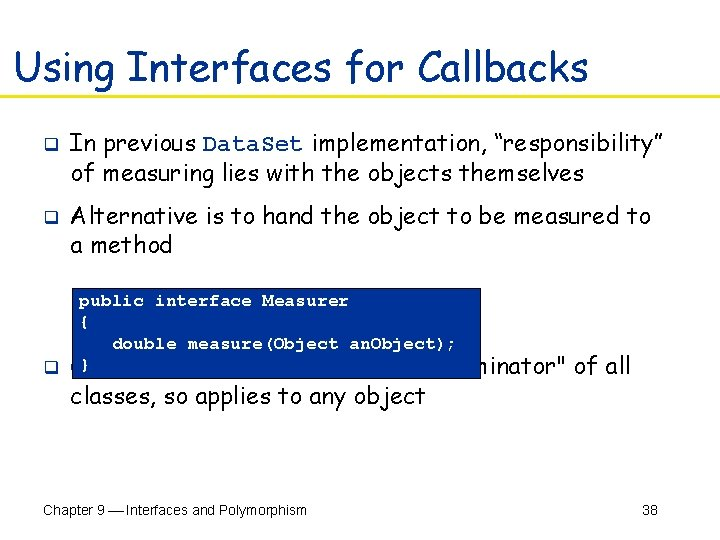Using Interfaces for Callbacks q q In previous Data. Set implementation, “responsibility” of measuring lies with the objects themselves Alternative is to hand the object to be measured to a method public interface Measurer { double measure(Object an. Object); } q Object is the "lowest common denominator" classes, so applies to any object Chapter 9 Interfaces and Polymorphism of all 38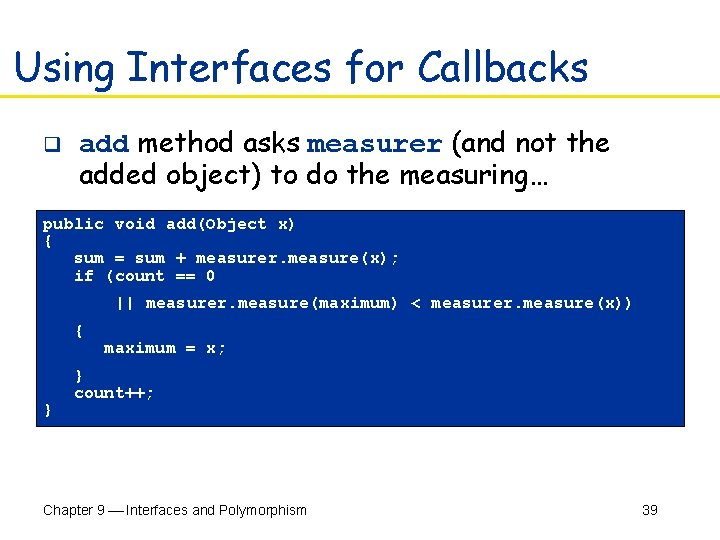Using Interfaces for Callbacks q add method asks measurer (and not the added object) to do the measuring… public void add(Object x) { sum = sum + measurer. measure(x); if (count == 0 || measurer. measure(maximum) < measurer. measure(x)) { maximum = x; } count++; } q …assuming measurer is of type Measurer Chapter 9 Interfaces and Polymorphism 39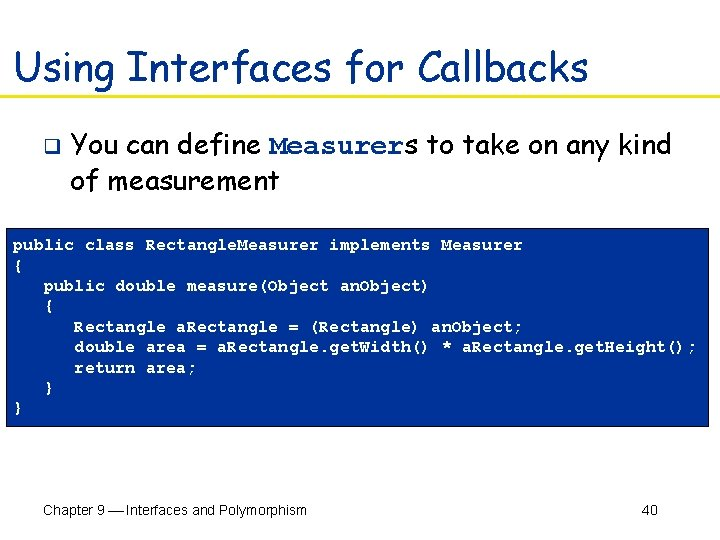Using Interfaces for Callbacks q You can define Measurers to take on any kind of measurement public class Rectangle. Measurer implements Measurer { public double measure(Object an. Object) { Rectangle a. Rectangle = (Rectangle) an. Object; double area = a. Rectangle. get. Width() * a. Rectangle. get. Height(); return area; } } Chapter 9 Interfaces and Polymorphism 40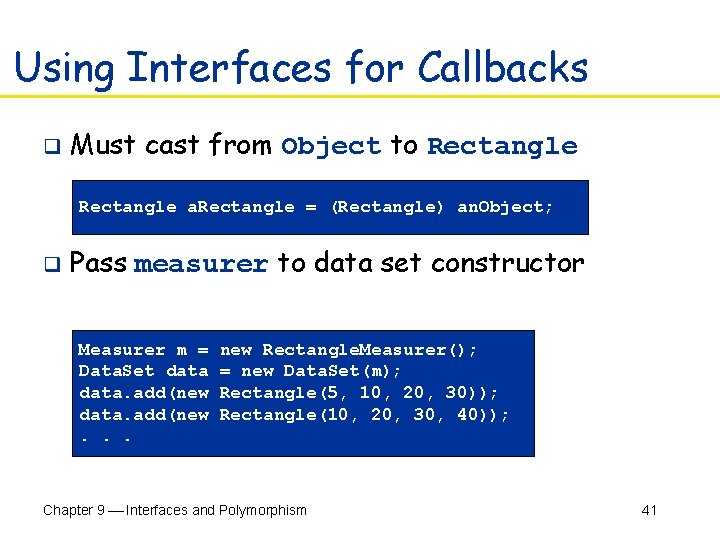Using Interfaces for Callbacks q Must cast from Object to Rectangle a. Rectangle = (Rectangle) an. Object; q Pass measurer to data set constructor Measurer m = new Rectangle. Measurer(); Data. Set data = new Data. Set(m); data. add(new Rectangle(5, 10, 20, 30)); data. add(new Rectangle(10, 20, 30, 40)); . . . Chapter 9 Interfaces and Polymorphism 41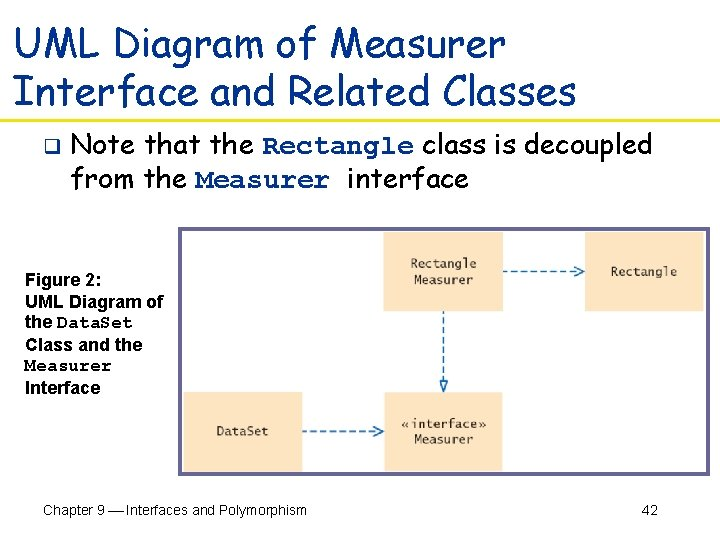UML Diagram of Measurer Interface and Related Classes q Note that the Rectangle class is decoupled from the Measurer interface Figure 2: UML Diagram of the Data. Set Class and the Measurer Interface Chapter 9 Interfaces and Polymorphism 42File Data. Set. java 01: /** 02: Computes the average of a set of data values. 03: */ 04: public class Data. Set 05: { 06: /** 07: Constructs an empty data set with a given measurer. 08: @param a. Measurer measurer used to measure values 09: */ 10: public Data. Set(Measurer a. Measurer) 11: { 12: sum = 0; 13: count = 0; 14: maximum = null; 15: measurer = a. Measurer; 16: } 17: Continued… Chapter 9 Interfaces and Polymorphism 43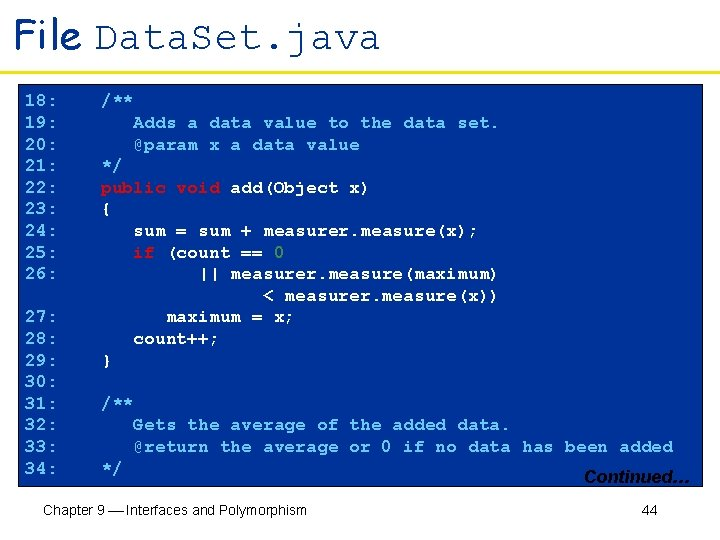File Data. Set. java 18: /** 19: Adds a data value to the data set. 20: @param x a data value 21: */ 22: public void add(Object x) 23: { 24: sum = sum + measurer. measure(x); 25: if (count == 0 26: || measurer. measure(maximum) < measurer. measure(x)) 27: maximum = x; 28: count++; 29: } 30: 31: /** 32: Gets the average of the added data. 33: @return the average or 0 if no data has been added 34: */ Continued… Chapter 9 Interfaces and Polymorphism 44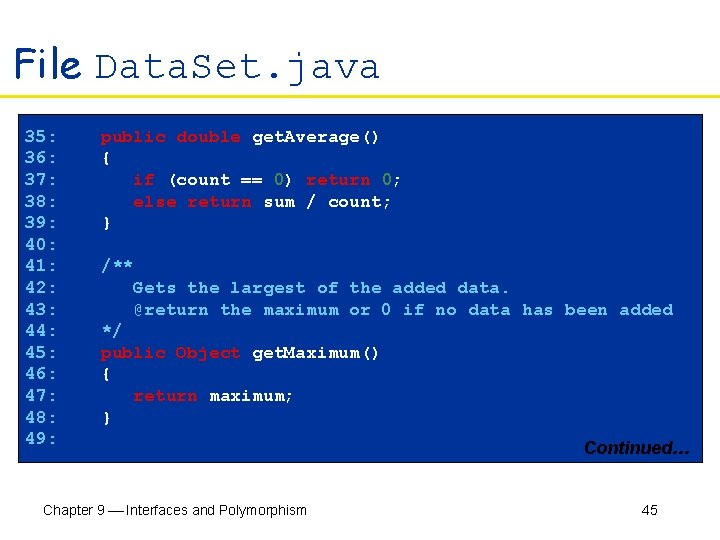File Data. Set. java 35: public double get. Average() 36: { 37: if (count == 0) return 0; 38: else return sum / count; 39: } 40: 41: /** 42: Gets the largest of the added data. 43: @return the maximum or 0 if no data has been added 44: */ 45: public Object get. Maximum() 46: { 47: return maximum; 48: } 49: Continued… Chapter 9 Interfaces and Polymorphism 45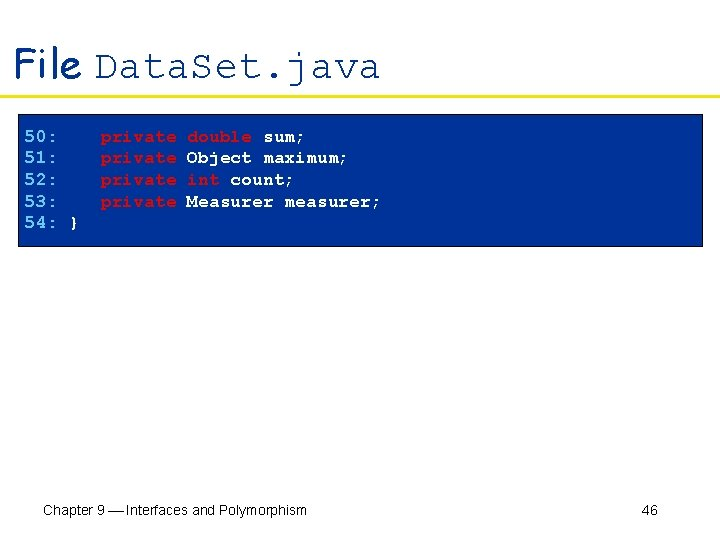File Data. Set. java 50: private double sum; 51: private Object maximum; 52: private int count; 53: private Measurer measurer; 54: } Chapter 9 Interfaces and Polymorphism 46File Data. Set. Tester 2. java 01: import java. awt. Rectangle; 02: 03: /** 04: This program demonstrates the use of a Measurer. 05: */ 06: public class Data. Set. Tester 2 07: { 08: public static void main(String[] args) 09: { 10: Measurer m = new Rectangle. Measurer(); 11: 12: Data. Set data = new Data. Set(m); 13: 14: data. add(new Rectangle(5, 10, 20, 30)); 15: data. add(new Rectangle(10, 20, 30, 40)); 16: data. add(new Rectangle(20, 30, 5, 10)); 17: Continued… Chapter 9 Interfaces and Polymorphism 47File Data. Set. Tester 2. java 18: System. out. println("Average area = " + data. get. Average()); 19: Rectangle max = (Rectangle) data. get. Maximum(); 20: System. out. println("Maximum area rectangle = " + max); 21: } 22: } Chapter 9 Interfaces and Polymorphism 48File Measurer. java 01: /** 02: Describes any class whose objects can measure other objects. 03: */ 04: public interface Measurer 05: { 06: /** 07: Computes the measure of an object. 08: @param an. Object the object to be measured 09: @return the measure 10: */ 11: double measure(Object an. Object); 12: } Chapter 9 Interfaces and Polymorphism 49File Rectangle. Measurer. java 01: import java. awt. Rectangle; 02: 03: /** 04: Objects of this class measure rectangles by area. 05: */ 06: public class Rectangle. Measurer implements Measurer 07: { 08: public double measure(Object an. Object) 09: { 10: Rectangle a. Rectangle = (Rectangle) an. Object; 11: double area = a. Rectangle. get. Width() * a. Rectangle. get. Height(); 12: return area; 13: } 14: } 15: Continued… Chapter 9 Interfaces and Polymorphism 50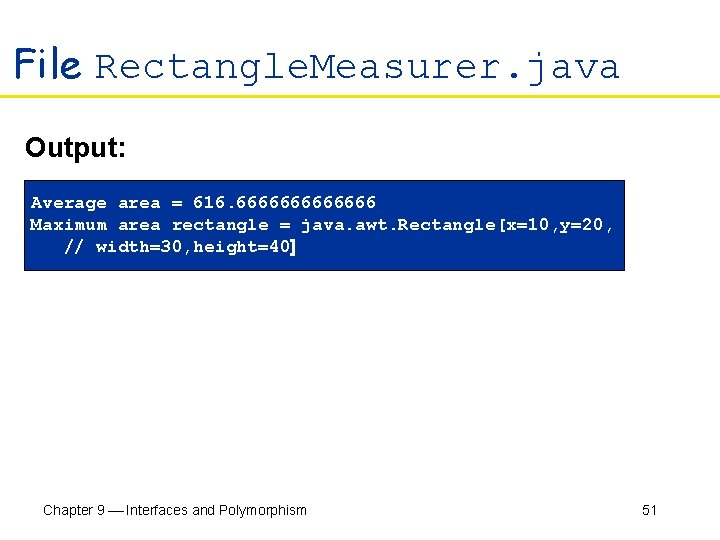File Rectangle. Measurer. java Output: Average area = 616. 6666666 Maximum area rectangle = java. awt. Rectangle[x=10, y=20, // width=30, height=40] Chapter 9 Interfaces and Polymorphism 51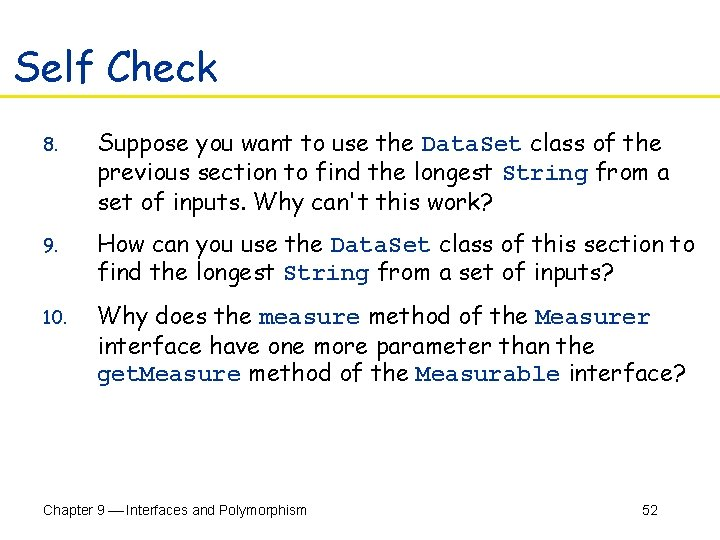Self Check 8. Suppose you want to use the Data. Set class of the previous section to find the longest String from a set of inputs. Why can't this work? 9. How can you use the Data. Set class of this section to find the longest String from a set of inputs? 10. Why does the measure method of the Measurer interface have one more parameter than the get. Measure method of the Measurable interface? Chapter 9 Interfaces and Polymorphism 52Answers 8. The String class doesn't implement the Measurable interface 9. Implement a class String. Measurer that implements the Measurer interface (i. e. , use callback) 10. A measurer measures an object, whereas get. Measure measures "itself", that is, the implicit parameter Chapter 9 Interfaces and Polymorphism 53Inner Classes q Trivial class can be defined inside a method public class Data. Set. Tester 3 { public static void main(String[] args) { class Rectangle. Measurer implements Measurer { . . . } Measurer m = new Rectangle. Measurer(); Data. Set data = new Data. Set(m); . . . } } Continued… Chapter 9 Interfaces and Polymorphism 54Inner Classes q q If inner class is defined inside an enclosing class, but outside its methods, it is available to all methods of enclosing class Compiler turns an inner class into a regular class file Data. Set. Tester\$1\$Rectangle. Measurer. class Chapter 9 Interfaces and Polymorphism 55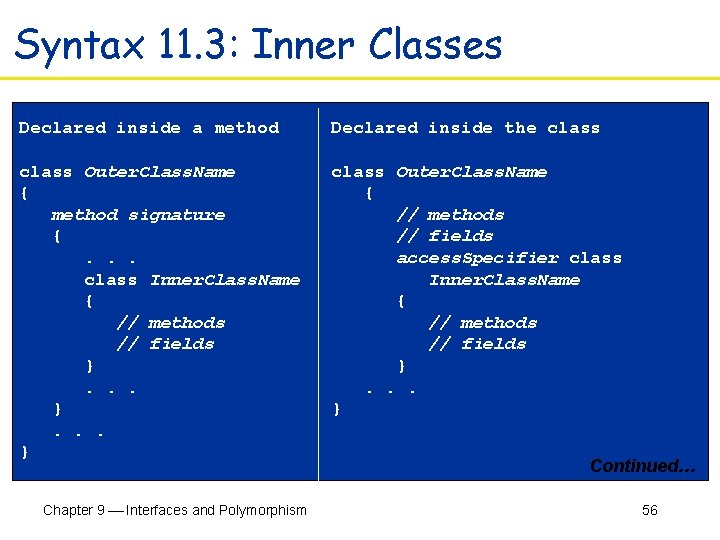Syntax 11. 3: Inner Classes Declared inside a method Declared inside the class Outer. Class. Name { method signature { . . . class Inner. Class. Name { // methods // fields } . . . } class Outer. Class. Name { // methods // fields access. Specifier class Inner. Class. Name { // methods // fields } . . . } Chapter 9 Interfaces and Polymorphism Continued… 56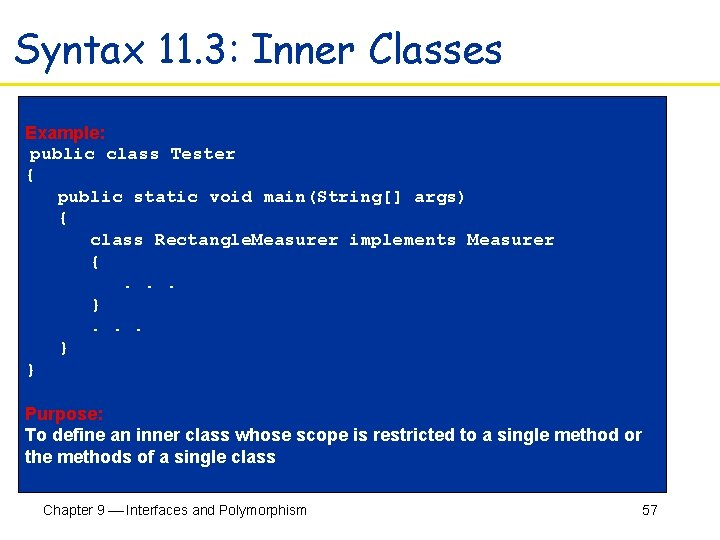Syntax 11. 3: Inner Classes Example: public class Tester { public static void main(String[] args) { class Rectangle. Measurer implements Measurer { . . . } . . . } } Purpose: To define an inner class whose scope is restricted to a single method or the methods of a single class Chapter 9 Interfaces and Polymorphism 57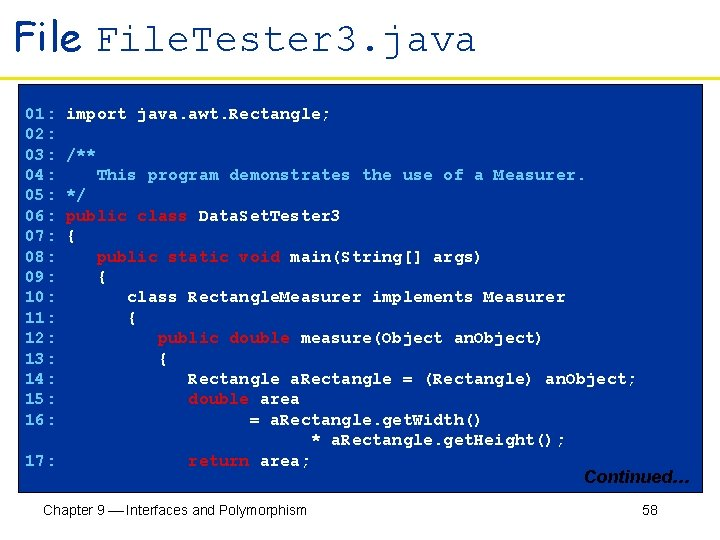File. Tester 3. java 01: import java. awt. Rectangle; 02: 03: /** 04: This program demonstrates the use of a Measurer. 05: */ 06: public class Data. Set. Tester 3 07: { 08: public static void main(String[] args) 09: { 10: class Rectangle. Measurer implements Measurer 11: { 12: public double measure(Object an. Object) 13: { 14: Rectangle a. Rectangle = (Rectangle) an. Object; 15: double area 16: = a. Rectangle. get. Width() * a. Rectangle. get. Height(); 17: return area; Continued… Chapter 9 Interfaces and Polymorphism 58File. Tester 3. java 18: } 19: } 20: 21: Measurer m = new Rectangle. Measurer(); 22: 23: Data. Set data = new Data. Set(m); 24: 25: data. add(new Rectangle(5, 10, 20, 30)); 26: data. add(new Rectangle(10, 20, 30, 40)); 27: data. add(new Rectangle(20, 30, 5, 10)); 28: 29: System. out. println("Average area = " + data. get. Average()); 30: Rectangle max = (Rectangle) data. get. Maximum(); 31: System. out. println("Maximum area rectangle = " + max); 32: } 33: } Chapter 9 Interfaces and Polymorphism 59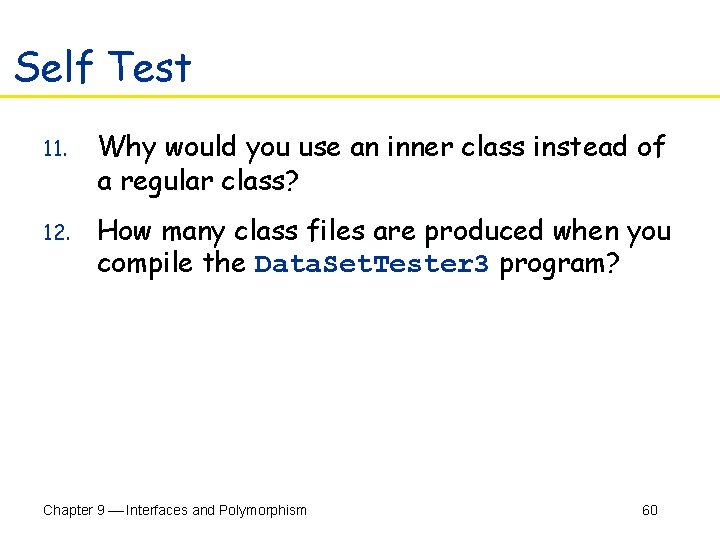Self Test 11. Why would you use an inner class instead of a regular class? 12. How many class files are produced when you compile the Data. Set. Tester 3 program? Chapter 9 Interfaces and Polymorphism 60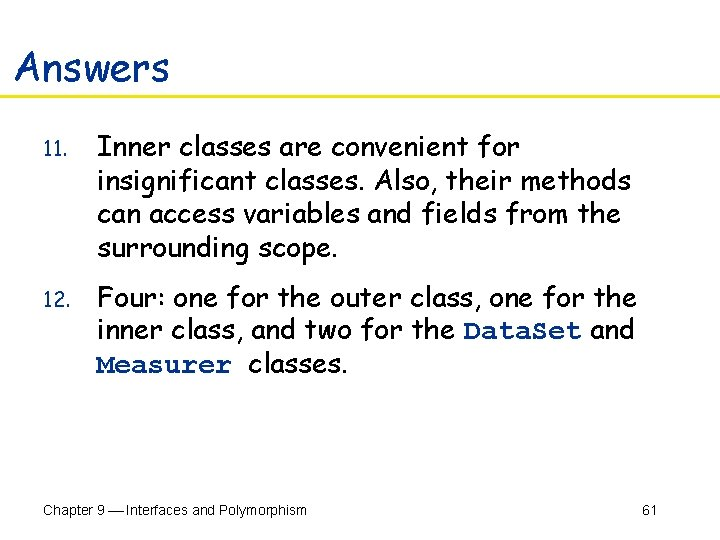Answers 11. Inner classes are convenient for insignificant classes. Also, their methods can access variables and fields from the surrounding scope. 12. Four: one for the outer class, one for the inner class, and two for the Data. Set and Measurer classes. Chapter 9 Interfaces and Polymorphism 61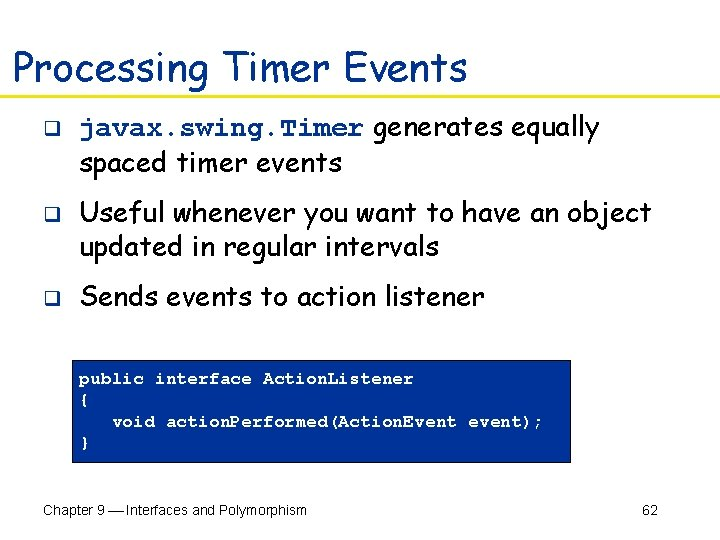Processing Timer Events q q q javax. swing. Timer generates equally spaced timer events Useful whenever you want to have an object updated in regular intervals Sends events to action listener public interface Action. Listener { void action. Performed(Action. Event event); } Chapter 9 Interfaces and Polymorphism 62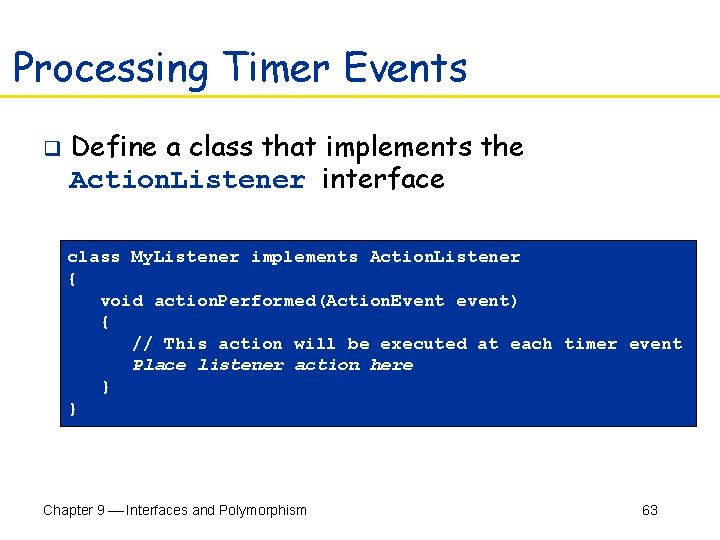Processing Timer Events q Define a class that implements the Action. Listener interface class My. Listener implements Action. Listener { void action. Performed(Action. Event event) { // This action will be executed at each timer event Place listener action here } } Chapter 9 Interfaces and Polymorphism 63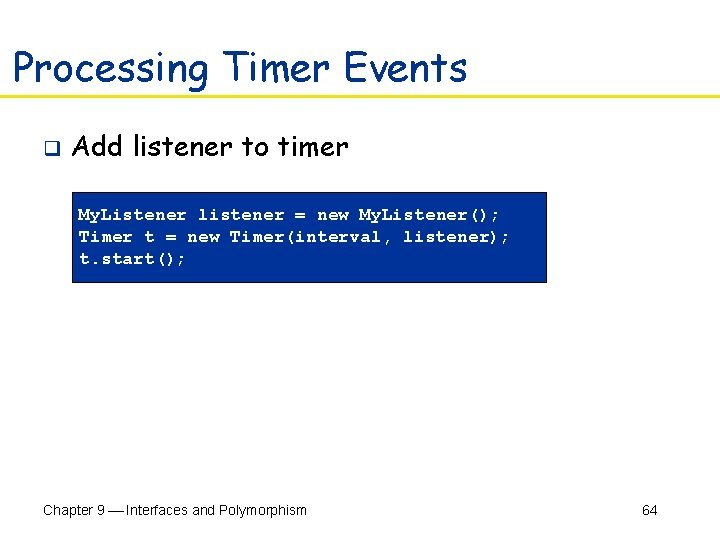Processing Timer Events q Add listener to timer My. Listener listener = new My. Listener(); Timer t = new Timer(interval, listener); t. start(); Chapter 9 Interfaces and Polymorphism 64Example: Countdown q Example: a timer that counts down to zero Figure 3: Running the Time. Tester Program Chapter 9 Interfaces and Polymorphism 65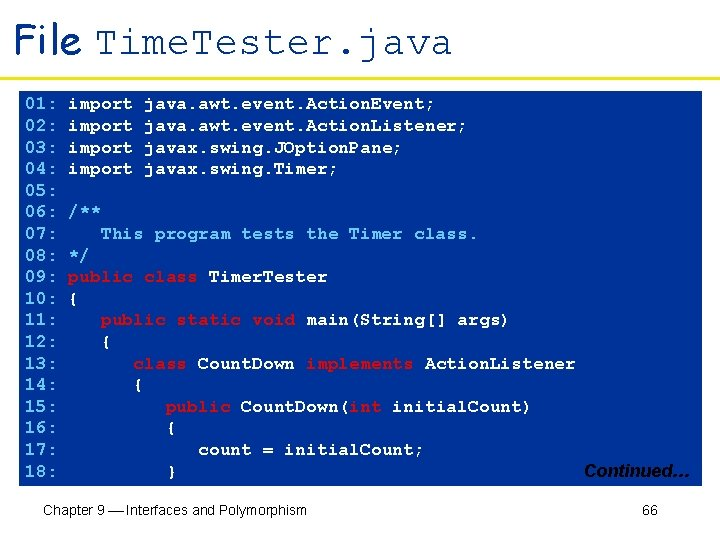File Time. Tester. java 01: import java. awt. event. Action. Event; 02: import java. awt. event. Action. Listener; 03: import javax. swing. JOption. Pane; 04: import javax. swing. Timer; 05: 06: /** 07: This program tests the Timer class. 08: */ 09: public class Timer. Tester 10: { 11: public static void main(String[] args) 12: { 13: class Count. Down implements Action. Listener 14: { 15: public Count. Down(int initial. Count) 16: { 17: count = initial. Count; 18: } Continued… Chapter 9 Interfaces and Polymorphism 66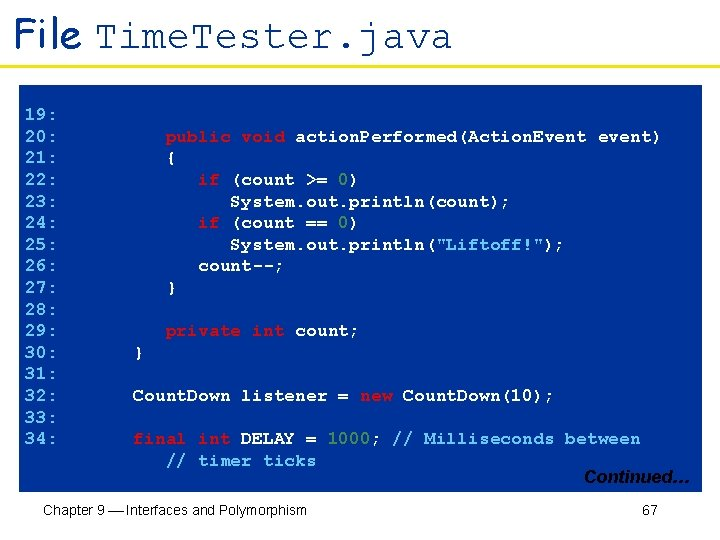File Time. Tester. java 19: 20: public void action. Performed(Action. Event event) 21: { 22: if (count >= 0) 23: System. out. println(count); 24: if (count == 0) 25: System. out. println("Liftoff!"); 26: count--; 27: } 28: 29: private int count; 30: } 31: 32: Count. Down listener = new Count. Down(10); 33: 34: final int DELAY = 1000; // Milliseconds between // timer ticks Continued… Chapter 9 Interfaces and Polymorphism 67File Time. Tester. java 35: Timer t = new Timer(DELAY, listener); 36: t. start(); 37: 38: JOption. Pane. show. Message. Dialog(null, "Quit? "); 39: System. exit(0); 40: } 41: } Chapter 9 Interfaces and Polymorphism 68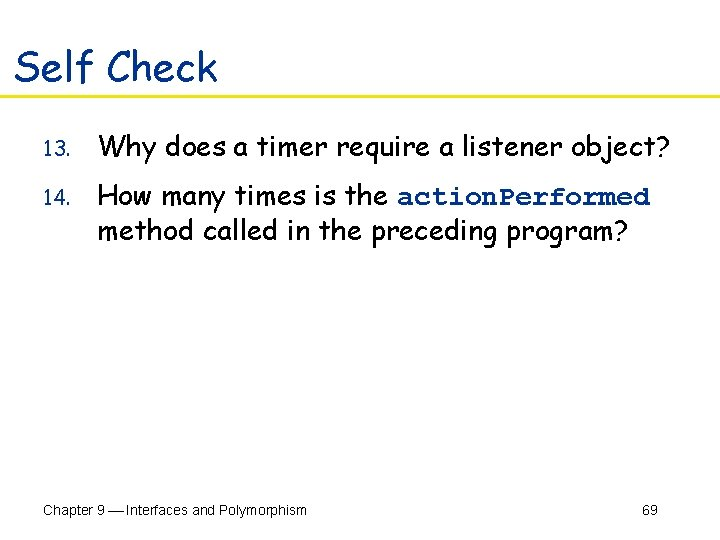Self Check 13. Why does a timer require a listener object? 14. How many times is the action. Performed method called in the preceding program? Chapter 9 Interfaces and Polymorphism 69Answers 13. The timer needs to call some method whenever the time interval expires. It calls the action. Performed method of the listener object. 14. It depends. The method is called once per second. The first eleven times, it prints a message. The remaining times, it exits silently. The timer is only terminated when the user quits the program. Chapter 9 Interfaces and Polymorphism 70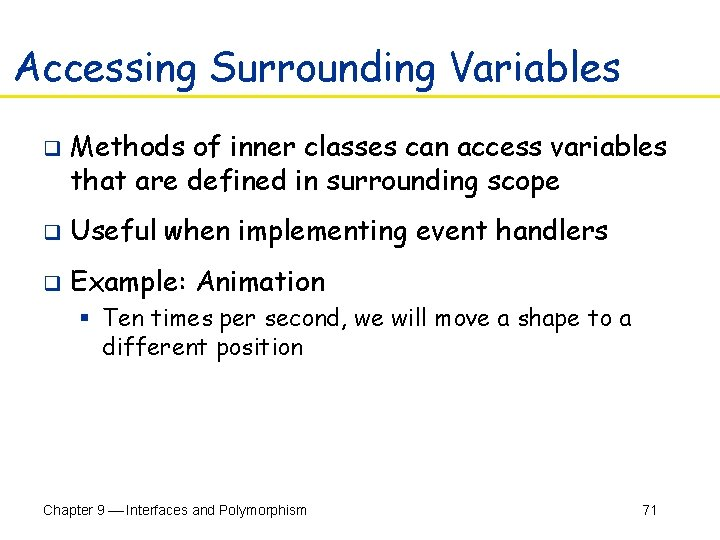Accessing Surrounding Variables q Methods of inner classes can access variables that are defined in surrounding scope q Useful when implementing event handlers q Example: Animation § Ten times per second, we will move a shape to a different position Chapter 9 Interfaces and Polymorphism 71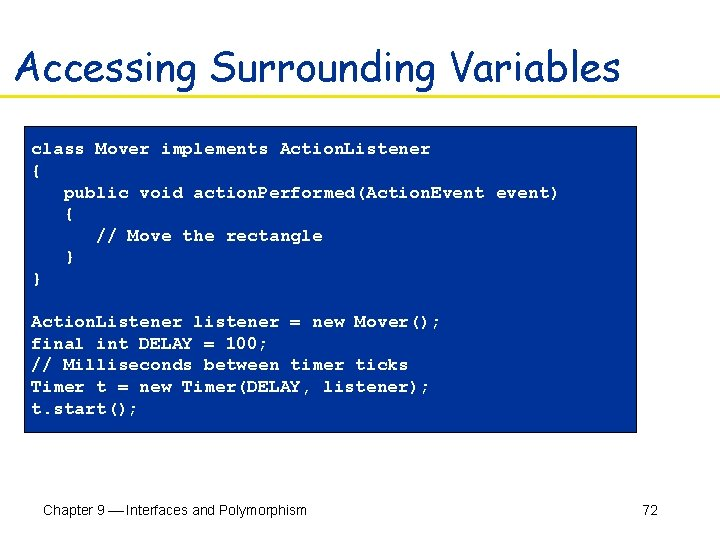Accessing Surrounding Variables class Mover implements Action. Listener { public void action. Performed(Action. Event event) { // Move the rectangle } } Action. Listener listener = new Mover(); final int DELAY = 100; // Milliseconds between timer ticks Timer t = new Timer(DELAY, listener); t. start(); Chapter 9 Interfaces and Polymorphism 72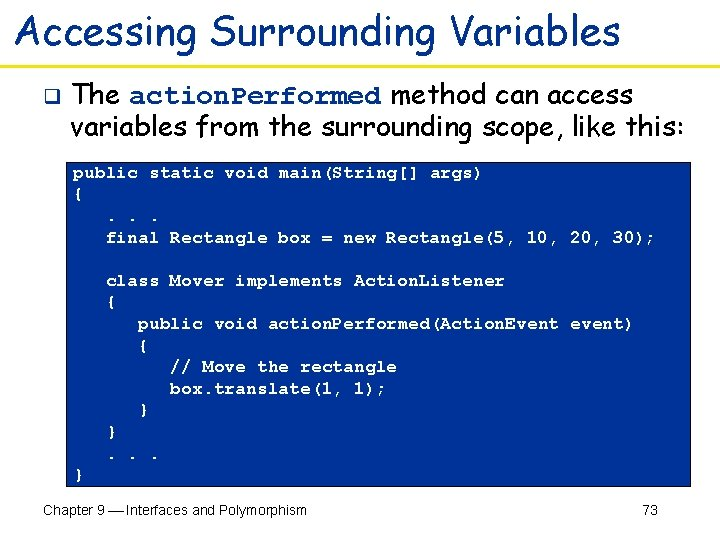Accessing Surrounding Variables q The action. Performed method can access variables from the surrounding scope, like this: public static void main(String[] args) { . . . final Rectangle box = new Rectangle(5, 10, 20, 30); class Mover implements Action. Listener { public void action. Performed(Action. Event event) { // Move the rectangle box. translate(1, 1); } } . . . } Chapter 9 Interfaces and Polymorphism 73Accessing Surrounding Variables q q q Local variables that are accessed by an innerclass method must be declared as final Inner class can access fields of surrounding class that belong to the object that constructed the inner class object An inner class object created inside a static method can only access static surrounding fields Chapter 9 Interfaces and Polymorphism 74File Time. Tester 2. java 01: import java. awt. Rectangle; 02: import java. awt. event. Action. Event; 03: import java. awt. event. Action. Listener; 04: import javax. swing. JOption. Pane; 05: import javax. swing. Timer; 06: 07: /** 08: This program uses a timer to move a rect once per second. 09: */ 10: public class Timer. Tester 2 11: { 12: public static void main(String[] args) 13: { 14: final Rectangle box = new Rectangle(5, 10, 20, 30); 15: 16: class Mover implements Action. Listener 17: { Continued… Chapter 9 Interfaces and Polymorphism 75File Time. Tester 2. java 18: public void action. Performed(Action. Event event) 19: { 20: box. translate(1, 1); 21: System. out. println(box); 22: } 23: } 24: 25: Action. Listener listener = new Mover(); 26: 27: final int DELAY = 100; // Milliseconds between ticks 28: Timer t = new Timer(DELAY, listener); 29: t. start(); 30: 31: JOption. Pane. show. Message. Dialog(null, "Quit? "); 32: System. out. println("Last box position: " + box); 33: System. exit(0); 34: } 35: } Chapter 9 Interfaces and Polymorphism 76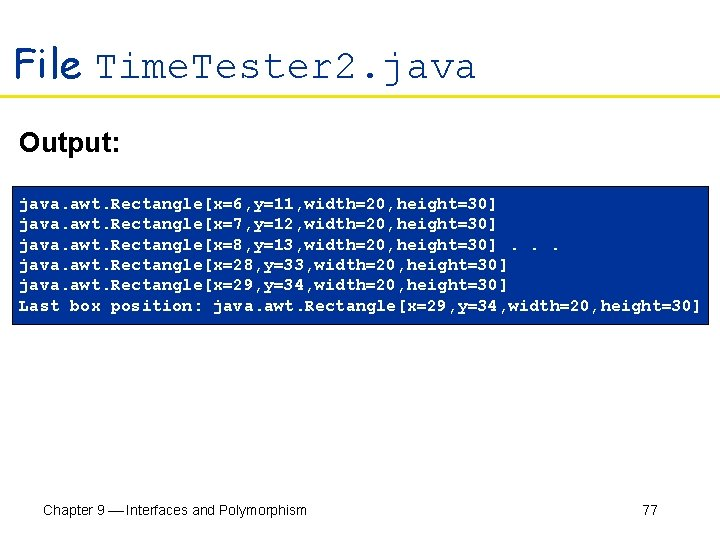File Time. Tester 2. java Output: java. awt. Rectangle[x=6, y=11, width=20, height=30] java. awt. Rectangle[x=7, y=12, width=20, height=30] java. awt. Rectangle[x=8, y=13, width=20, height=30]. . . java. awt. Rectangle[x=28, y=33, width=20, height=30] java. awt. Rectangle[x=29, y=34, width=20, height=30] Last box position: java. awt. Rectangle[x=29, y=34, width=20, height=30] Chapter 9 Interfaces and Polymorphism 77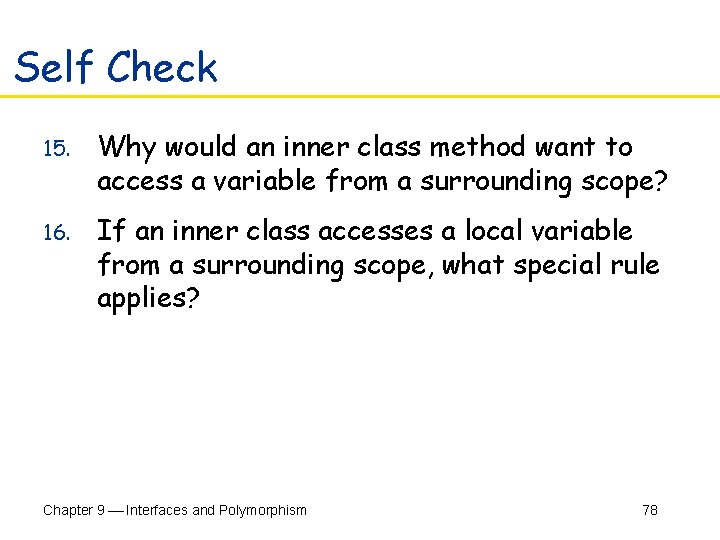Self Check 15. Why would an inner class method want to access a variable from a surrounding scope? 16. If an inner class accesses a local variable from a surrounding scope, what special rule applies? Chapter 9 Interfaces and Polymorphism 78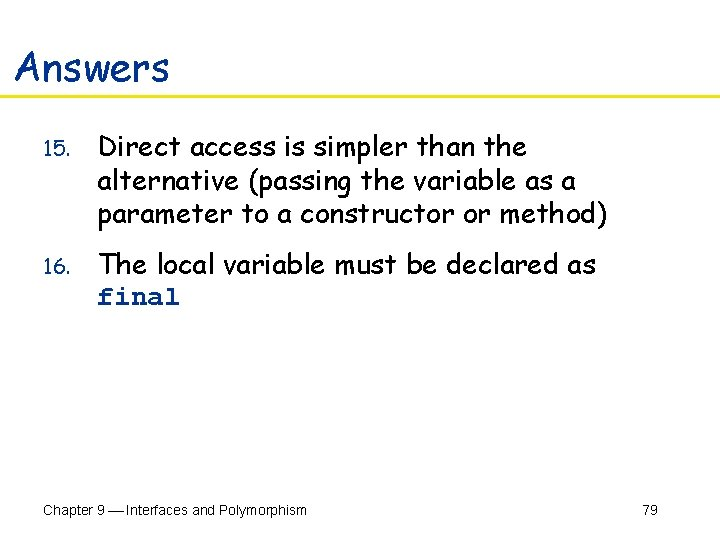Answers 15. Direct access is simpler than the alternative (passing the variable as a parameter to a constructor or method) 16. The local variable must be declared as final Chapter 9 Interfaces and Polymorphism 79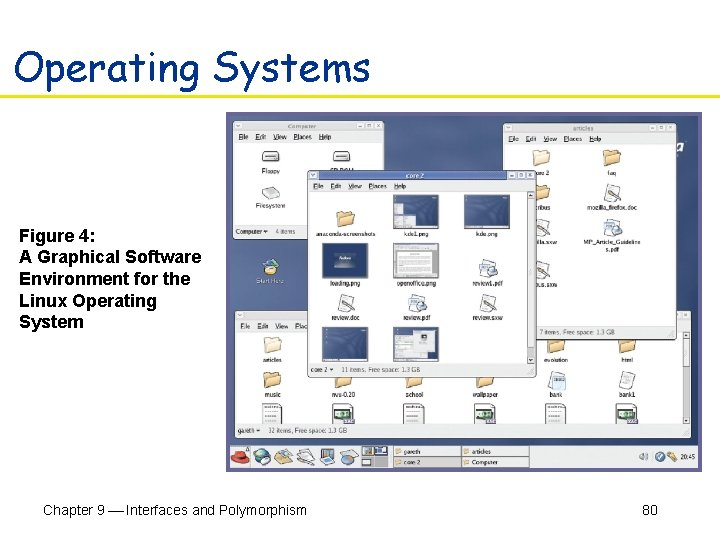Operating Systems Figure 4: A Graphical Software Environment for the Linux Operating System Chapter 9 Interfaces and Polymorphism 80